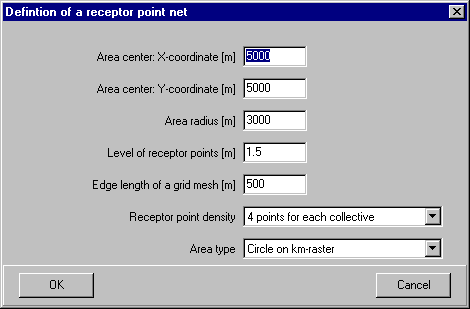# P&K ODOR

## Definition of a receptor point net### Area center: X/Y-Coordinates

The center coordinates of a receptor point area is to be set here.

The radius of a receptor point area is to be set here. Only areas which are completely in a circle within this radius around the area center, will be used in the creation of evaluation areas.

### Receptor point level

If the concentration array is generated with vertical distribution, a height of the receptor point area can be entered. Otherwise the level of the concentration array will be selected automatically.

### Edge length of a grid mesh

The receptor point area is built up with collectives (evaluation areas), which are arranged in quadratic areas with this edge length. An edge length of 1000 m complies with the procedure in the TA Luft.

### Receptor point density

A square area can contain 4 receptor points (one on each corner) or additional 5 points in the middle of the edge length between the corners and in the center. Also collectives with only one point per area (in the center) can be produced. The resulting characteristic quantities calculated are point values (not area values).

### Area type

Select from the following types:

• Circle on km-raster
• Circle around center of area
• Rectangle on km-raster
• Rectangle around center of area

Language: